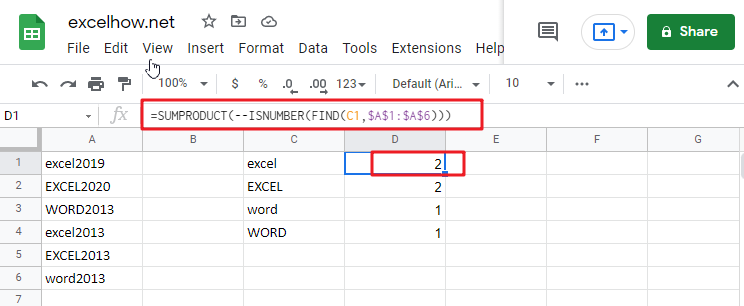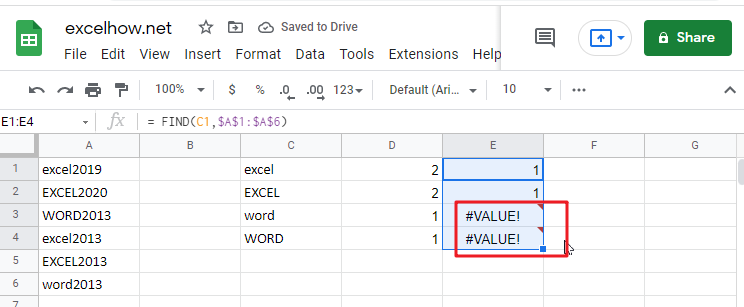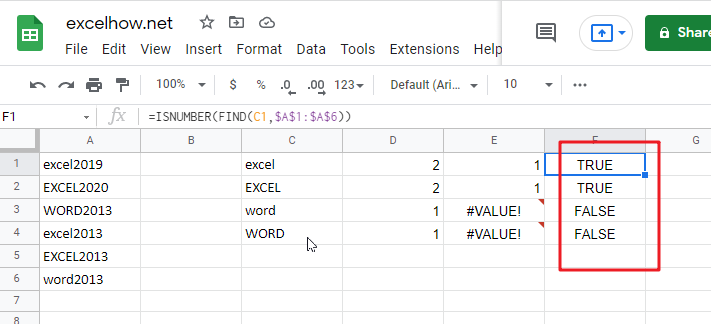# Count Cells that are Case Sensitive in Google SheetsSimple generic formula:

`=SUMPRODUCT(--ISNUMBER(FIND(value, range)))`

## Summary

You may use a formula depending on the ISNUMBER and FIND functions and the SUMPRODUCT function to calculate the number of cells that contain specified text (i.e., hold a substring), taking into consideration upper and lower case. D1 in the example displayed has the following formula, which has been written down:

`=SUMPRODUCT(--ISNUMBER(FIND(C1,\$A\$1:\$A\$6)))`

## Let’s See How This Formula Works

In this example, the purpose is to count the number of times each code appears as a substring in a case-sensitive manner, as shown below. When calculating text values, the functions COUNTIF and COUNTIFS are excellent choices. However, since these functions are not case-sensitive, they cannot be utilized to address this issue. To check for substrings, the FIND function should be used in conjunction with the ISNUMBER function, and the SUMPRODUCT function should be used to sum up, the results.

`= FIND(C1,\$A\$1:\$A\$6)`

The FIND function is case-sensitive and accepts three parameters: find text, within the text, and start num. It is also case-sensitive and takes three arguments. Here is the text we are searching for, and this is the text that we are searching inside. Start num is the number of characters to begin searching in find text. Because start num defaults to 1, we are not supplying a number in this instance. This is necessary since we always want FIND to begin with the first character. When finding the text is discovered inside within text, FIND returns the position of the found text as a number: When finding the text is located within text, FIND returns the position of the found text as a number:Note: When the find text function fails to yield a result, FIND returns the #VALUE! Error.

`=ISNUMBER(FIND(C1,\$A\$1:\$A\$6))`

This implies that we may be using the ISNUMBER function to transform the output of the FIND function into a TRUE or FALSE value using the ISNUMBER function. A TRUE value will arise from any number, while a FALSE value will result from any error:As demonstrated in the example, we have four substrings in column C and a variety of codes in columns A1:A6, representing the data for the designated range. Specifically, we want to know how many times each substring in C1:C4 occurs in A1:A6, and we want to know how many times each substring is case-sensitive.

### Related Functions

• Excel ISNUMBER function
The Excel ISNUMBER function returns TRUE if the value in a cell is a numeric value, otherwise it will return FALSE.The syntax of the ISNUMBER function is as below:= ISNUMBER (value)…
The Google Sheets FIND function returns the position of the first text string (sub string) within another text string.The syntax of the FIND function is as below:= FIND(find_text, within_text,[start_num])…
The Google Sheets SUMPRODUCT function multiplies corresponding components in the given one or more arrays or ranges, and returns the sum of those products.The syntax of the SUMPRODUCT function is as below:= SUMPRODUCT (array1,[array2],…)…
The Google Sheets CHOOSE function returns a value from a list of values based on index. The syntax of the CHOOSE function is as below:=CHOOSE (index_num, value1,[value2],…) …
The Google SheetsCOUNTIFS function returns the count of cells in a range that meet one or more criteria. The syntax of the COUNTIFS function is as below:= COUNTIFS(criteria_range1, criteria1, [criteria_range2, criteria2]…)…
Related Posts

Rank Data with Multiple Criteria in google sheets

If you are an enthusiastic Google Sheets user, then you may have an urgent task to rank a specified datelist based on given multiple criteria; you can easily perform this task manually, which is also feasible, but it only works ...

Rank Numeric Values with Duplicate in Google Sheets

Suppose you have an urgent task of ranking a list with duplicate values, what would be the first approach you would think of? Is there a quick and easy way to do this task in Google Sheets? This article will ...

Calculate Compound Interest using FV Function in Google Sheets

This article will show you how to calculate compound interest in Google Sheets. It will help you calculate the interest you will accrue on a given amount of money. There are several ways to calculate compound interest Google Sheets. One ...

Count Attendance and Absence with Google Sheets COUNTIF

In our campus life and work life, we usually record everyone's attendance. Today we will introduce you the application of Google Sheets COUNTIF function to count the attendance. Using a week as an example, we use the following example to ...

Find the Closest Data to the Data Provided in Google Sheets

In our daily work, we may encounter such an issue that to find the closest value to a certain value. In fact, google sheets internal functions can help us solve this problem. In today’s article, we will show you how ...

Basic Array Formula With Examples in Google Sheets

Are you searching for an article for getting the basic array formula with different examples for better understanding? Then congratulations because you have just landed on the right article. In this article, you would get to know the basic array ...

Average Of Numbers With Multiple Criteria in Google Sheets

Have you ever come across a task to calculate the average of the numbers with respect to multiple criteria in google sheets? Are you tired of doing this cumbersome task manually? Are you willing to do this task smartly in ...

Calculate Average Of Last 5 Or N Values In Columns in Google Sheets

Suppose you come across a task where you need to calculate the average of the last 2 or 3 numeric values in google sheets, then what would you do? If you are new to google sheets, then your first attempt ...

Find And Retrieve Missing Values in Google Sheets

Find and retrieve is a common basic operation in Google Sheets for tables. In out daily work, we may encounter this situation that some data was lost after several operations on a table in Google Sheets. In fact, we can ...

Calculating Average Of The Numbers in Google Sheets

Are you weary of investing a lot of time and effort in manually calculating the average of the numbers by including or excluding 0 and calculating the average of the top 3 scores? Then congratulations because you have just landed ...

Sidebar